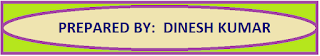### Resource Centre Mathematics

Resource Centre Mathematics Mathematics worksheet, mathematics basic points and formulas, mathematics lesson plan, mathematics multiple choice questions Workplace Dashboard CBSE Syllabus For Session 2023-24 For  :   Classes IX & X    |   Classes XI & XII Watch Videos on Maths Solutions CLASS IX MATHEMATICS FORMULAS &  BASIC CONCEPTS

## Mathematics Assignment Class IX Chapter 3 Coordinate Geometry

Mathematics Assignment for Class IX Ch -3, Co-ordinate Geometry, strictly according to the CBSE syllabus. Math worksheet based on the topic Co-ordinate Geometry.

Question 1:

What  is Cartesian coordinate system

Answer: A system which involves the x-axis and y-axis on the two dimensional surface is called a cartesian coordinate system.

Question 2:

What we call the horizontal line in Cartesian coordinate system.

Answer: Horizontal line is called the x - axis.

Question 3:

What we call the vertical  line in Cartesian coordinate system.

Answer: Vertical Line is called the y - axis.

Question 4:

Which point is called the origin and what are its coordinates.

Answer: The point of intersection of x - axis and y - axis is called the Origin. Coordinates of origin are (0,0)

Question 5:

What is the point on x – axis.

Answer: Any point on the x - axis is (a, 0)

Question 6:

What is the point on y-axis.

Answer: Any point on the y - axis is (0, b)

Question 7:

What do you mean by ordinates.

Answer: Point on y - axis is called ordinate.

Question 8:

What do you mean by abscissa.

Answer: Point on the x-axis is called abscissa.

Question 9:

Write the coordinates of the points given in the following graph. Also write In which quadrant or on which axis the following points lie.

A(3, 4) lie in I quadrant

B(-4, 3) lie in II quadrant

C(-2. -3) lie in III quadrant

D(3, -2) lie in IV quadrant

E(2, 0) lie on the x-axis

F(-2, 0) lie on the x-axis

G(0, 2) lie on y-axis

H(0, -2) lie on y-axis

Question 10:

Write the abscissa and ordinate of the following points

(-3, 5),   (0, 9),   (-5, 0),   (3,3),   (7, -9),  (12, 13)

Abscissa:  -3, 0, -5, 3, 7, 12

Ordinate:  5, 9, 0, 3, -9, 13

Question 11:

Write the coordinates of the point :

a) Whose ordinate is -5 and which lies on y- axis.

b) Which lies on x-axis and y – axes both.

c) Whose abscissa is -3 and which lies on x – axis.

a) (0,-5)

b) Point on the x-axis is (a, 0) and Point on the y-axis is (0, b)

c) (-3, 0)

Question 12:

Write the coordinates of the points given in the following graph. Also write In which quadrant or on which axis the following points lie.

Question 13:

What is the perpendicular distance of the point (3, 4) from

a)  x – axis        b) Y – axis

a) 4 unit   b) 3 unit

Question 14:

What is the perpendicular distance of the point (7, 26) from

a)  x – axis        b) Y – axis

a) 26 unit   b) 7 unit

Question 15:

Name the quadrant in which abscissa and ordinate have different sign.

Ans: In II and IV quadrant abscissa and ordinate have different sign.

Question 16:

Plot the points P(1,0), Q(4,0), and S(1, 3). Find the coordinates of point R such that PQRS is a square. Also find the area of the square.

Coordinates of R(4, 3),  Area of square PQRS = 9 square units)

Question 17:

Mark the points A(2, 2), B(2, -2), C(-2, -2) and D(-2, 2) on the graph paper and join these points in order. Identify the figure so obtained. Also find the area of the figure.

Square, Area = 16cm2]

Question 18:

Plot the points P(-1, 0), Q(0, 1) and R(2, 3) on the graph paper and check whether they are collinear or not.

Answer: If P, Q, R all points lie on a straight line then points are collinear.

Question 19:

If the points A(0, -6), B(0, 2 and C (a, 3) lie on the y-axis, then find the value of a.

Answer: For points on the y-axis the abscissa is "0" So the value of a = 0

Question 20:

From the given graph write :

a) The coordinates of the points B and F

b) The abscissas of points D and H

c) The ordinates of the points A and C

d) The perpendicular distance of the point G from x-axis

a)  B(-5, -4), F(6, 1)
b) D(1, 1),  H(0, 4)
c) A(-4, 1),  C(-2, 0)
d) G(6, 4) , Perpendicular distance of poin G from x-axis is 4

##Download Complete PDF File

1.It is very helpful for students.